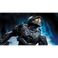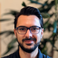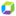cancel
Showing results for
Show  only  | Search instead for
Did you mean:

This product reached the end of support date on March 31, 2021.

## Measure count and SumGuide

Is it possible to Add the count of multiple measure together for Sum and divide by another measure count?

For example i have created measures and BTs. I want to add 3 measures (# of transactions) together and divide by a 4th measure (# of transactions). Is this possible? Can i do this within a business transaction?

3 REPLIES 3Dynatrace Pro

Hi Malik,

There is no measure for such operations. We only offer the Calculation -> Rate measure which allows you to divide one measure by another (i.e. to obtain percentage).

If your first 3 measures are just transaction counts, maybe you can create a BT where the filter has entries for all 3 measures, all with logical operator "OR". Then your count will be under one measure, but for all 3 types of transactions.

Then you can use the Calculation > Rate measure and divide this by the 4th measure.

Regards,